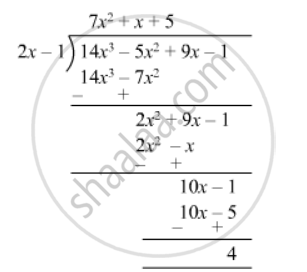Advertisement Remove all ads

# Divide 14x3 − 5x2 + 9x − 1 by 2x − 1 and Find the Quotient and Remainder - Mathematics

Sum

Divide 14x3 − 5x2 + 9x − 1 by 2x − 1 and find the quotient and remainder

Advertisement Remove all ads

#### Solution$\text{Quotient} = 7 x^2 + x + 5$
$\text{Remainder} = 4$

Is there an error in this question or solution?
Advertisement Remove all ads

#### APPEARS IN

RD Sharma Class 8 Maths
Chapter 8 Division of Algebraic Expressions
Exercise 8.4 | Q 16 | Page 11
Advertisement Remove all ads

#### Video TutorialsVIEW ALL 

Advertisement Remove all ads
Share
Notifications

View all notifications

Forgot password?
Course